# 1. 基本语法

## 正则表达式语法规则

\ 将下一字符标记为特殊字符、文本、反向引用或八进制转义符。例如，"n"匹配字符"n"。"\n"匹配换行符。序列"\\"匹配"\"，"\("匹配"("。
^ 匹配输入字符串开始的位置。如果设置了 RegExp 对象的 Multiline 属性，^ 还会与"\n"或"\r"之后的位置匹配。
$匹配输入字符串结尾的位置。如果设置了 RegExp 对象的 Multiline 属性，$ 还会与"\n"或"\r"之前的位置匹配。
* 零次或多次匹配前面的字符或子表达式。例如，zo* 匹配"z"和"zoo"。* 等效于 {0,}。
+ 一次或多次匹配前面的字符或子表达式。例如，"zo+"与"zo"和"zoo"匹配，但与"z"不匹配。+ 等效于 {1,}。
? 零次或一次匹配前面的字符或子表达式。例如，"do(es)?"匹配"do"或"does"中的"do"。? 等效于 {0,1}。
{n} n 是非负整数。正好匹配 n 次。例如，"o{2}"与"Bob"中的"o"不匹配，但与"food"中的两个"o"匹配。
{n,} n 是非负整数。至少匹配 n 次。例如，"o{2,}"不匹配"Bob"中的"o"，而匹配"foooood"中的所有 o。"o{1,}"等效于"o+"。"o{0,}"等效于"o*"。
{n,m} mn 是非负整数，其中 n <= m。匹配至少 n 次，至多 m 次。例如，"o{1,3}"匹配"fooooood"中的头三个 o。'o{0,1}' 等效于 'o?'。注意：您不能将空格插入逗号和数字之间。
? 当此字符紧随任何其他限定符（、+、?、{n}、{n,}、{n,m*}）之后时，匹配模式是"非贪心的"。"非贪心的"模式匹配搜索到的、尽可能短的字符串，而默认的"贪心的"模式匹配搜索到的、尽可能长的字符串。例如，在字符串"oooo"中，"o+?"只匹配单个"o"，而"o+"匹配所有"o"。
. 匹配除"\r\n"之外的任何单个字符。若要匹配包括"\r\n"在内的任意字符，请使用诸如"[\s\S]"之类的模式。
(pattern) 匹配 pattern 并捕获该匹配的子表达式。可以使用 $0…$9 属性从结果"匹配"集合中检索捕获的匹配。若要匹配括号字符 ( )，请使用"("或者")"。
(?:pattern) 匹配 pattern 但不捕获该匹配的子表达式，即它是一个非捕获匹配，不存储供以后使用的匹配。这对于用"or"字符 (|) 组合模式部件的情况很有用。例如，'industr(?:y|ies) 是比 'industry|industries' 更经济的表达式。
(?=pattern) 执行正向预测先行搜索的子表达式，该表达式匹配处于匹配 pattern 的字符串的起始点的字符串。它是一个非捕获匹配，即不能捕获供以后使用的匹配。例如，'Windows (?=95|98|NT|2000)' 匹配"Windows 2000"中的"Windows"，但不匹配"Windows 3.1"中的"Windows"。预测先行不占用字符，即发生匹配后，下一匹配的搜索紧随上一匹配之后，而不是在组成预测先行的字符后。
(?!pattern) 执行反向预测先行搜索的子表达式，该表达式匹配不处于匹配 pattern 的字符串的起始点的搜索字符串。它是一个非捕获匹配，即不能捕获供以后使用的匹配。例如，'Windows (?!95|98|NT|2000)' 匹配"Windows 3.1"中的 "Windows"，但不匹配"Windows 2000"中的"Windows"。预测先行不占用字符，即发生匹配后，下一匹配的搜索紧随上一匹配之后，而不是在组成预测先行的字符后。
x|y 匹配 xy。例如，'z|food' 匹配"z"或"food"。'(z|f)ood' 匹配"zood"或"food"。
[xyz] 字符集。匹配包含的任一字符。例如，"[abc]"匹配"plain"中的"a"。
[^xyz] 反向字符集。匹配未包含的任何字符。例如，"[^abc]"匹配"plain"中"p"，"l"，"i"，"n"。
[a-z] 字符范围。匹配指定范围内的任何字符。例如，"[a-z]"匹配"a"到"z"范围内的任何小写字母。
[^a-z] 反向范围字符。匹配不在指定的范围内的任何字符。例如，"[^a-z]"匹配任何不在"a"到"z"范围内的任何字符。
\b 匹配一个字边界，即字与空格间的位置。例如，"er\b"匹配"never"中的"er"，但不匹配"verb"中的"er"。
\B 非字边界匹配。"er\B"匹配"verb"中的"er"，但不匹配"never"中的"er"。
\cx 匹配 x 指示的控制字符。例如，\cM 匹配 Control-M 或回车符。x 的值必须在 A-Z 或 a-z 之间。如果不是这样，则假定 c 就是"c"字符本身。
\d 数字字符匹配。等效于 [0-9]。
\D 非数字字符匹配。等效于 [^0-9]。
\f 换页符匹配。等效于 \x0c 和 \cL。
\n 换行符匹配。等效于 \x0a 和 \cJ。
\r 匹配一个回车符。等效于 \x0d 和 \cM。
\s 匹配任何空白字符，包括空格、制表符、换页符等。与 [ \f\n\r\t\v] 等效。
\S 匹配任何非空白字符。与 [^ \f\n\r\t\v] 等效。
\t 制表符匹配。与 \x09 和 \cI 等效。
\v 垂直制表符匹配。与 \x0b 和 \cK 等效。
\w 匹配任何字类字符，包括下划线。与"[A-Za-z0-9_]"等效。
\W 与任何非单词字符匹配。与"[^A-Za-z0-9_]"等效。
\xn 匹配 n，此处的 n 是一个十六进制转义码。十六进制转义码必须正好是两位数长。例如，"\x41"匹配"A"。"\x041"与"\x04"&"1"等效。允许在正则表达式中使用 ASCII 代码。
\num 匹配 num，此处的 num 是一个正整数。到捕获匹配的反向引用。例如，"(.)\1"匹配两个连续的相同字符。
\n 标识一个八进制转义码或反向引用。如果 *n* 前面至少有 n 个捕获子表达式，那么 n 是反向引用。否则，如果 n 是八进制数 (0-7)，那么 n 是八进制转义码。
\nm 标识一个八进制转义码或反向引用。如果 *nm* 前面至少有 nm 个捕获子表达式，那么 nm 是反向引用。如果 *nm* 前面至少有 n 个捕获，则 n 是反向引用，后面跟有字符 m。如果两种前面的情况都不存在，则 *nm* 匹配八进制值 nm，其中 nm 是八进制数字 (0-7)。
\nml n 是八进制数 (0-3)，ml 是八进制数 (0-7) 时，匹配八进制转义码 nml
\un 匹配 n，其中 n 是以四位十六进制数表示的 Unicode 字符。例如，\u00A9 匹配版权符号 (©)。

## 1.2 java 语法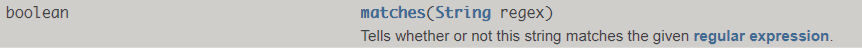### 1.2.1 String类的正则函数

• replaceALL
• split
• matches(最重要)

#### replaceAll的用法

String replaceAll(String regex, String replacement)
Replaces each substring of this string that matches the given regular expression with the given replacement.
@Test
public void TestThre(){
System.out.println("UUID.randomUUID().toString() = " + UUID.randomUUID().toString());
System.out.println("UUID.randomUUID().toString().replaceAll(\"-\", \"\") = " + UUID.randomUUID().toString().replaceAll("-", ""));
}


UUID.randomUUID().toString() = 1ed154bd-3725-49ae-ab31-8d92f2e76774
UUID.randomUUID().toString().replaceAll("-", "") = f321fd646b664655bd4100aee4214c38


#### split用法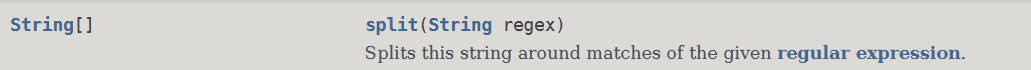@Test
public void testSplit(){
String[] strings = "asdf_fsg shteh gkrm_grw4h feg".split("(_|\\s)");
for (String string : strings) {
System.out.println(string);
}
}


asdf
fsg
shteh
gkrm
grw4h
feg


#### matches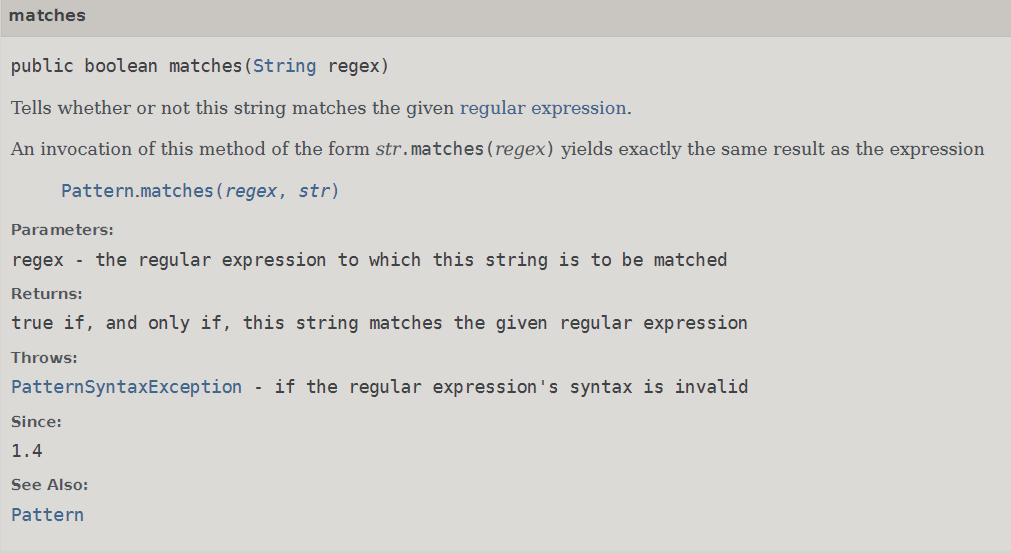@Test
public void testMatchers(){
System.out.println("第一种方法");
System.out.println("12345@qust.edu.com.cn".matches("\\d{3,}@[\\w\\.]+edu\\.com\\.cn"));
System.out.println("第二种方法");
System.out.println(Pattern.matches("\\d{3,}@[\\w\\.]+edu\\.com\\.cn", "12345@qust.edu.com.cn"));
System.out.println("第三种方法");
System.out.println(Pattern.compile("\\d{3,}@[\\w\\.]+edu\\.com\\.cn").matcher("12345@qust.edu.com.cn").matches());

}


• str.matches(regex)
• Pattern.matches(regex,str)
• Pattern.compile(regex).matcher(str)

### 1.2.2 Pattern和Matcher

java.util.regex是一个用正则表达式所订制的模式来对字符串进行匹配工作的类库包。它包括两个类：Pattern和Matcher Pattern 一个Pattern是一个正则表达式经编译后的表现模式。 Matcher 一个Matcher对象是一个状态机器，它依据Pattern对象做为匹配模式对字符串展开匹配检查。 首先一个Pattern实例订制了一个所用语法与PERL的类似的正则表达式经编译后的模式，然后一个Matcher实例在这个给定的Pattern实例的模式控制下进行字符串的匹配工作。

Pattern和Matcher

Pattern pattern1 = Pattern.compile("(\\d+)((?i)[a-z]+)");
Matcher matcher = pattern1.matcher("1234awdHf");
while (matcher.find()) {
System.out.println("matcher.group(1) = " + matcher.group(1));
System.out.println("matcher.group(2) = " + matcher.group(2));
System.out.println("matcher.group() = " + matcher.group());
}


### 1.2.3 断言

https://cloud.tencent.com/developer/article/1149481

https://www.aqee.net/post/regular-expression-to-match-string-not-containing-a-word.html

#### 1.2.3.1 情景导入

//检测由空格开始的词汇
Pattern pattern4 = Pattern.compile("\\s(?i)\\w+");
Matcher matcher3 = pattern4.matcher(" hello world");
while (matcher3.find()) {
String group = matcher3.group();
//可以用下面这种方法去除空格
System.out.println("group.trim() = " + group.trim());
//            group.trim() = hello
//            group.trim() = world
}


#### 1.2.3.3 断言的语法规则

(?=X) X, via zero-width positive lookahead 零宽前行正向断言
(?!X) X, via zero-width negative lookahead 零宽前行负向断言
(?<=X) X, via zero-width positive lookbehind 零宽后行正向断言
(?<!X) X, via zero-width negative lookbehind 零宽后行负向断言

#### 1.2.3.4 零宽断言为什么叫零宽断言

##### 前行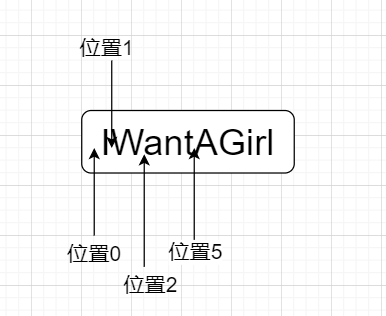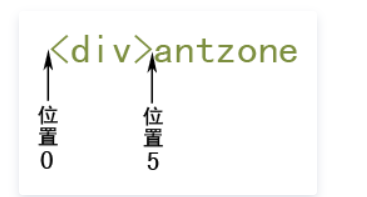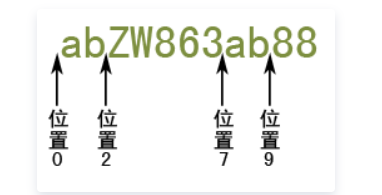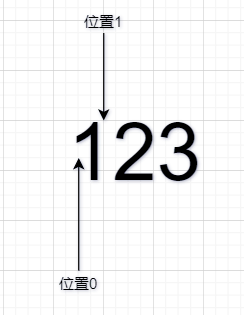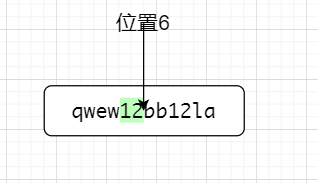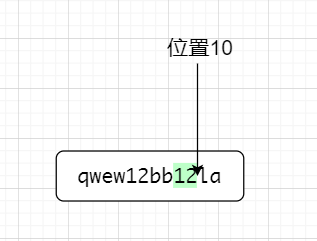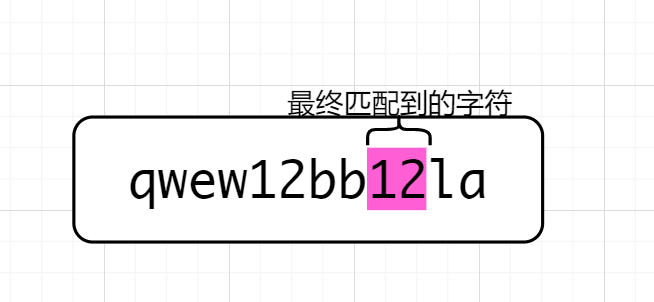##### 负向

(?=X) X, via zero-width positive lookahead 零宽前行正向断言
(?!X) X, via zero-width negative lookahead 零宽前行负向断言
(?<=X) X, via zero-width positive lookbehind 零宽后行正向断言
(?<!X) X, via zero-width negative lookbehind 零宽后行负向断言

#### 1.2.3.5断言DEMO

• 后行正向断言,该断言匹配前面是aa的字符

//后行正向断言
Pattern compile = Pattern.compile("(?<=aa)\\w+");
Matcher matcher = compile.matcher(" aadfds fdgs daafs");
while (matcher.find()) {
System.out.println(matcher.group());//dfds
}


👆运行结果为👇

dfds
fs

• 后行负向断言,该断言匹配的是前面不是$的数字 Pattern compile1 = Pattern.compile("(?<!\\$|\\d)\\d+");
Matcher matcher1 = compile1.matcher("$14 23");//23 while (matcher1.find()) { System.out.println("matcher1.group() = " + matcher1.group()); }  👆运行结果为👇 matcher1.group() = 23  我来解释一下，正则表达式(?<!\\$|\\d)\\d+,断言的意思是不匹配前面为$或数字的数字。 模拟一下匹配的过程，也许会更加明白是怎么一回事儿，先说一下"$14 23"，位置我就不在图中标了，自己数一下吧👇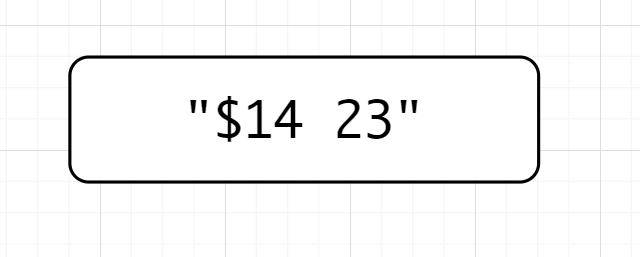(?<!\\$|\\d)\\d+先匹配数字，匹配到了1，位置是1，因为断言是不占宽度的。因为是后行，检测到左边是$,不符合断言要求，就来到了位置2(1和4之间)，位置二前面是数字不符合要求，类推，位置来到了2的前面，前面是空格，既不是数字也不是$，符合要求，故匹配23 • 先行正向断言 Matcher matcher2 = Pattern.compile("(?i)windows(?=2000|xp|10)").matcher("windowsXp"); while (matcher2.find()) { System.out.println("matcher2.group() = " + matcher2.group()); }  运行结果是👇  matcher2.group() = windows  我来稍微解释一下，"(?i)windows(?=2000|xp|10)" （?i）表明不区分大小写，Windows表明适配windows，(?=2000|xp|10)表明是零宽先行正向断言。我说一下匹配的过程，首先匹配windows，位置来到了windows后，零宽先行，后面是xp适配成功。 • 先行负向断言 Matcher matcher3 = Pattern.compile("(?i)Linux(?!12.3)").matcher("linux12."); while (matcher3.find()) { System.out.println("matcher3.group() = " + matcher3.group()); }  运行结果是👇 matcher3.group() = linux  这个就不解释了，自行理解吧 以上四个demo是最最基础的demo，在很多教程上对断言的讲解止步于此(实际上，很短教程连最基本的匹配过程都没讲，只讲了匹配的结果，这个要出大问题的)，务须掌握好上面的四个demo。 #### 1.2.3.6 断言的基础应用和实际用处 放一张思维导图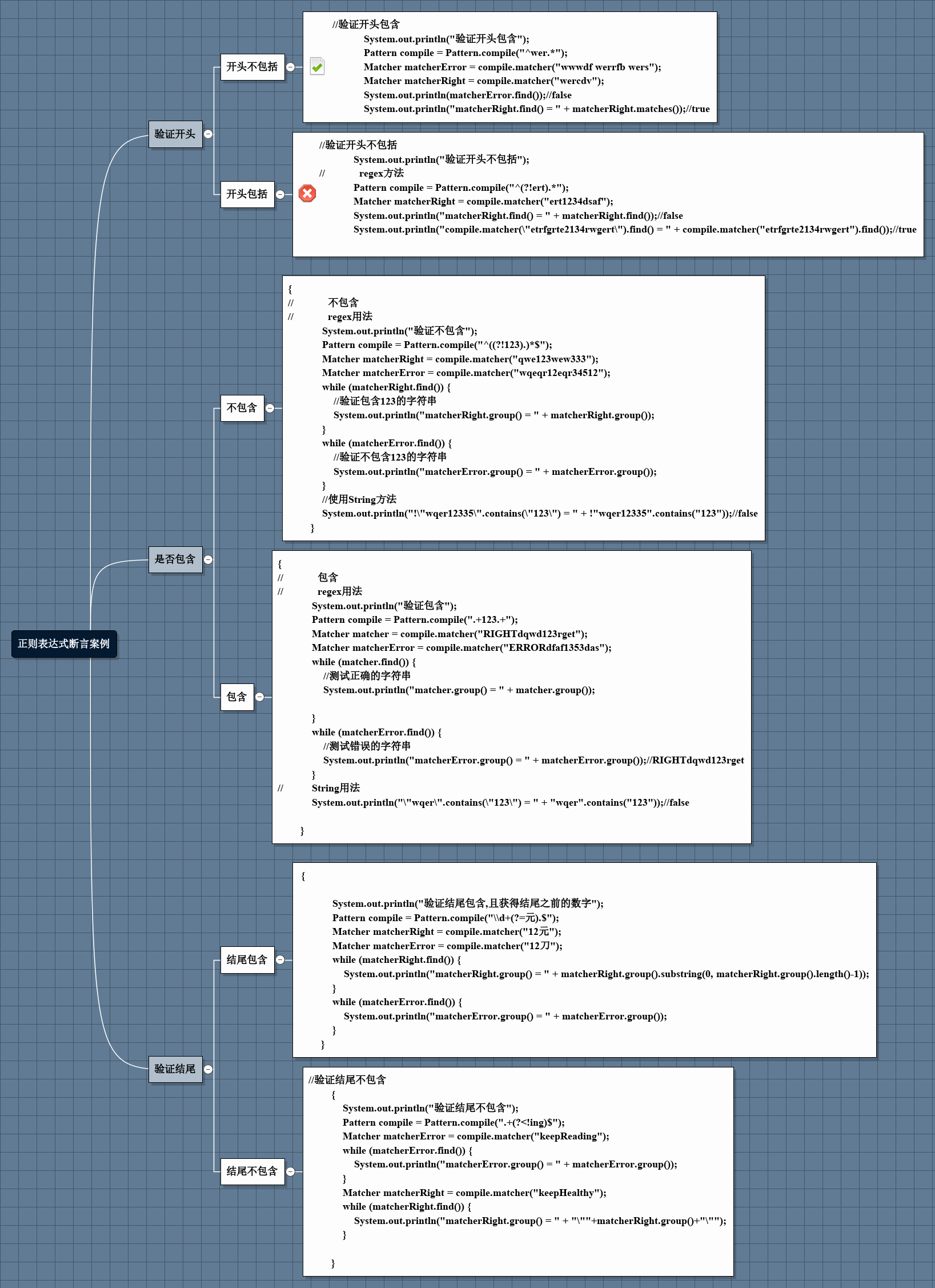这个我先说一下结论，在实际应用开发中，在应用诸如验证开头或结尾(只要求一行的开头或结尾)的实践中，大概有两种，一种是零宽，一种是非零宽。所谓零宽即匹配的数据不包括断言，非零宽反之，在某些情况下需要借助String方法的subString()方法。 一般情况下，使用断言，主要是用它零宽的属性，验证开头包含，结尾包含时一般不用断言，分组就能解决问题，当开头不包含或结尾不包含等问题时，使用断言是很好的办法。 演示三个👇 ##### 1.2.3.6.1 验证不包含 /* 不包含 */ { // 不包含 // regex用法 System.out.println("验证不包含"); Pattern compile = Pattern.compile("^((?!123).)*$");
Matcher matcherRight = compile.matcher("qwe123wew333");
Matcher matcherError = compile.matcher("wqeqr12eqr34512");
while (matcherRight.find()) {
//验证包含123的字符串
System.out.println("matcherRight.group() = " + matcherRight.group());
}
while (matcherError.find()) {
//验证不包含123的字符串
System.out.println("matcherError.group() = " + matcherError.group());//matcherError.group() = wqeqr12eqr34512
}
//使用String方法
System.out.println("!\"wqer12335\".contains(\"123\") = " + !"wqer12335".contains("123"));//!"wqer12335".contains("123") = false
}


验证不包含
matcherError.group() = wqeqr12eqr34512
!"wqer12335".contains("123") = false

##### 1.2.3.6.2 验证开头包含

{
System.out.println("验证开头包含");
Pattern compile = Pattern.compile("^(?=wer).*");
Matcher matcherError = compile.matcher("wwwdf werrfb wers");
Matcher matcherRight = compile.matcher("wercdv");
System.out.println(matcherError.find());//false
//            System.out.println("matcherRight.find() = " + matcherRight.find());//true
while (matcherRight.find()) {
System.out.println("matcherRight.group() = " + matcherRight.group());//wercdv
}
}


验证开头包含
false
matcherRight.group() = wercdv


^(?=wer).*匹配wercdv的时候，先因为^来到了位置0，位置0后面是符合.*的，遂开始匹配断言，注意这时候位置依然在位置0，因为是先行断言，位置0后面是wer符合断言要求，故匹配到了wercdv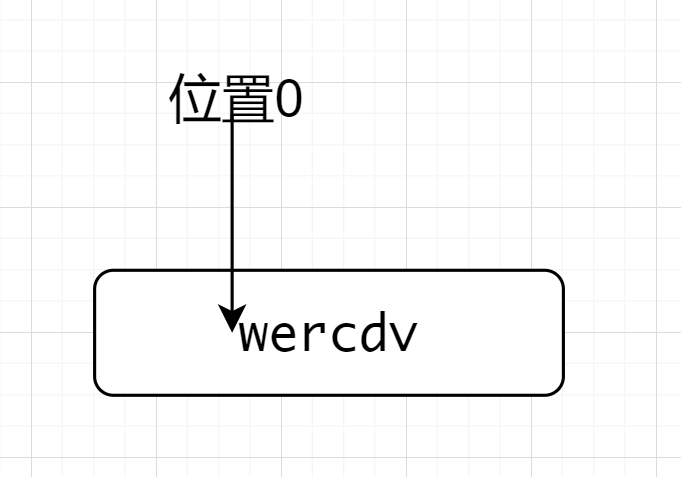##### 1.2.3.6.3 验证开头包含且匹配到的数据不包括断言

{
System.out.println("验证开头包含,匹配到的数据不包括断言");
Pattern compile = Pattern.compile("^wer(?<=wer)(.*)");
Matcher matcherError = compile.matcher("wwwdf werrfb wers");
Matcher matcherRight = compile.matcher("wercdv");
System.out.println(matcherError.find());//false

while (matcherRight.find()) {
System.out.println("matcherRight.start() = " + matcherRight.start());//0
System.out.println("matcherRight.end() = " + matcherRight.end());//6
System.out.println("matcherRight.group() = " + matcherRight.group(1));//cdv
}
}


验证开头包含,匹配到的数据不包括断言
false
matcherRight.start() = 0
matcherRight.end() = 6
matcherRight.group() = cdv


^wer(?<=wer)(.*)因为^所以先来到了位置0，然后匹配wer,来到了位置3，位置3后面符合.*的要求，因为后行匹配，前面是wer，符合要求，在取出的过程中，有一个分组，选取的是.*故最终取出cdv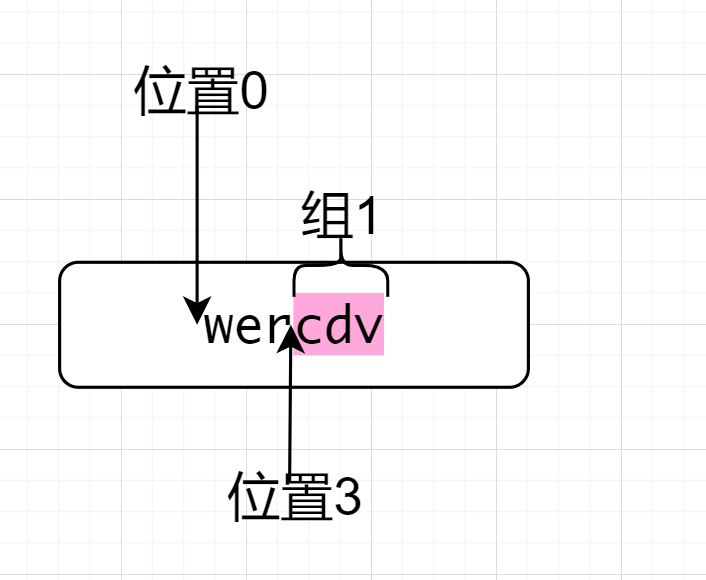##### 1.2.3.6.4 验证结尾包含，且匹配到的数据不包括断言

//验证结尾包含
{

System.out.println("验证结尾包含,且获得结尾之前的数字");
Pattern compile = Pattern.compile("(\\d+)(?=元).$"); Matcher matcherRight = compile.matcher("12元"); Matcher matcherError = compile.matcher("12刀"); while (matcherRight.find()) { System.out.println("matcherRight.group(1) = " + matcherRight.group(1));//12 } while (matcherError.find()) { System.out.println("matcherError.group() = " + matcherError.group()); } }  运行结果是👇 验证结尾包含,且获得结尾之前的数字 matcherRight.group(1) = 12  解析： (\\d+)(?=元).$因为$先来到位置3，如图所示👇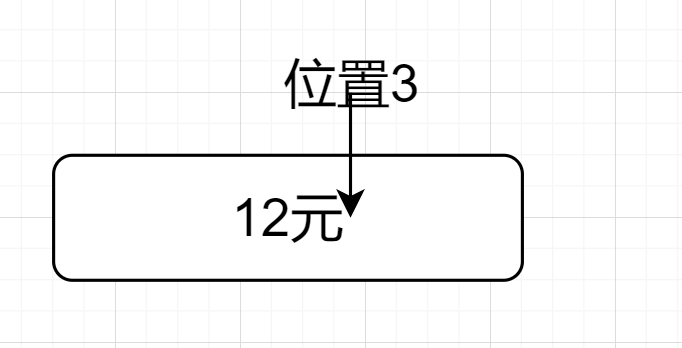然后因为(\\d+)(?=元).$.,位置向前一步，来到了位置2，然后前行断言，位置2的后面是元，前面是数字符合要求，如下图所示👇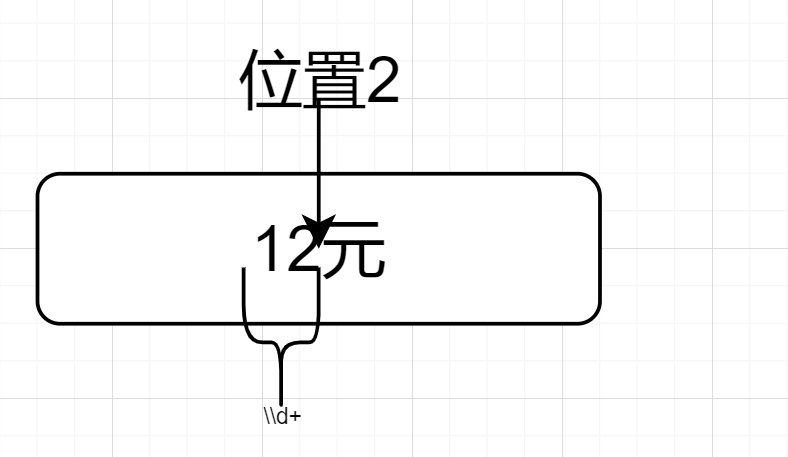##### 断言基础应用总体代码

/**
* 基础正则表达式实例
*/
@Test
public void testRegexOne() {
System.out.println(
"{\"name\": \"正则表达式相关断言有关的demo\",\n" +
"  " +
"\"个数\": 2" +
"}");
/*
包含
*/
{
//            包含
//            regex用法
System.out.println("验证包含");
Pattern compile = Pattern.compile(".+123.+");
Matcher matcher = compile.matcher("RIGHTdqwd123rget");
Matcher matcherError = compile.matcher("ERRORdfaf1353das");
while (matcher.find()) {
//测试正确的字符串
System.out.println("matcher.group() = " + matcher.group());

}
while (matcherError.find()) {
//测试错误的字符串
System.out.println("matcherError.group() = " + matcherError.group());//RIGHTdqwd123rget
}
//          String用法
System.out.println("\"wqer\".contains(\"123\") = " + "wqer".contains("123"));//false

}
/*
不包含
*/
{
//            不包含
//            regex用法
System.out.println("验证不包含");
Pattern compile = Pattern.compile("^((?!123).)*$"); Matcher matcherRight = compile.matcher("qwe123wew333"); Matcher matcherError = compile.matcher("wqeqr12eqr34512"); while (matcherRight.find()) { //验证包含123的字符串 System.out.println("matcherRight.group() = " + matcherRight.group()); } while (matcherError.find()) { //验证不包含123的字符串 System.out.println("matcherError.group() = " + matcherError.group());//matcherError.group() = wqeqr12eqr34512 } //使用String方法 System.out.println("!\"wqer12335\".contains(\"123\") = " + !"wqer12335".contains("123"));//!"wqer12335".contains("123") = false } /* 验证开头 */ //验证开头不包含 { //验证开头不包括 System.out.println("验证开头不包括"); // regex方法 Pattern compile = Pattern.compile("^(?!ert).*"); Matcher matcherRight = compile.matcher("ert1234dsaf"); // System.out.println("matcherRight.find() = " + matcherRight.find());//false while (matcherRight.find()) { System.out.println("matcherRight.group() = " + matcherRight.group()); } // System.out.println("compile.matcher(\"etrfgrte2134rwgert\").find() = " + compile.matcher("etrfgrte2134rwgert").find());//true Matcher matcherError = compile.matcher("etrfgrte2134rwgert"); while (matcherError.find()) { System.out.println("matcherError.group() = " + matcherError.group());//etrfgrte2134rwgert } } { System.out.println("验证开头包含"); Pattern compile = Pattern.compile("^(?=wer).*"); Matcher matcherError = compile.matcher("wwwdf werrfb wers"); Matcher matcherRight = compile.matcher("wercdv"); System.out.println(matcherError.find());//false // System.out.println("matcherRight.find() = " + matcherRight.find());//true while (matcherRight.find()) { System.out.println("matcherRight.group() = " + matcherRight.group());//wercdv } } //验证开头包含 { System.out.println("验证开头包含,匹配到的数据不包括断言"); Pattern compile = Pattern.compile("^wer(?<=wer)(.*)"); Matcher matcherError = compile.matcher("wwwdf werrfb wers"); Matcher matcherRight = compile.matcher("wercdv"); System.out.println(matcherError.find());//false while (matcherRight.find()) { System.out.println("matcherRight.start() = " + matcherRight.start());//0 System.out.println("matcherRight.end() = " + matcherRight.end());//6 System.out.println("matcherRight.group() = " + matcherRight.group(1));//cdv } } //验证结尾不包含 { System.out.println("验证结尾不包含"); Pattern compile = Pattern.compile(".+(?<!ing)$");
while (matcherError.find()) {
System.out.println("matcherError.group() = " + matcherError.group());
}
Matcher matcherRight = compile.matcher("keepHealthy");
while (matcherRight.find()) {

System.out.println("matcherRight.group() = " + "\""+matcherRight.group()+"\"");//"keepHealthy"
}

}
//验证结尾包含
{

System.out.println("验证结尾包含,且获得结尾之前的数字");
Pattern compile = Pattern.compile("(\\d+)(?=元).$"); Matcher matcherRight = compile.matcher("12元"); Matcher matcherError = compile.matcher("12刀"); while (matcherRight.find()) { System.out.println("matcherRight.group(1) = " + matcherRight.group(1));//12 } while (matcherError.find()) { System.out.println("matcherError.group() = " + matcherError.group()); } } }  #### 1.2.3.7 不按套路出牌，帮你彻底理解断言 一般情况下，使用断言，主要是用它零宽的属性，向验证开头包含，结尾包含时一般不用断言，分组就能解决问题，当开头不包含或结尾不包含等问题时，使用断言是很好的办法。 @Testpublic void testOther(){ System.out.println("不按套路来，帮您彻底理解断言"); Pattern compile = Pattern.compile("(?=re)\\w+"); Matcher matcher = compile.matcher("reading a book"); System.out.println("不按套路来的零宽先行正向断言"); while (matcher.find()) { System.out.println("matcher.group() = " + matcher.group());//matcher.group() = reading } System.out.println("不按套路来的零宽后行正向断言"); Pattern compile1 = Pattern.compile("\\w+(?i)(?<=re)"); Matcher matcher1 = compile1.matcher("i want toReading a book"); while (matcher1.find()) { System.out.println("matcher1.group() = " + matcher1.group());//matcher1.group() = toRe } System.out.println("不按套路来的零宽先行负向断言"); Pattern compile2 = Pattern.compile("(?i)ing(?!re)\\w+"); Matcher matcher2 = compile2.matcher("i am LovingReading a book and HatingBuy a book"); while (matcher2.find()) { System.out.println("matcher2.group() = " + matcher2.group());//matcher2.group() = ingBuy } System.out.println("不按套路来的零宽后行负向断言"); Pattern compile3 = Pattern.compile("(?i)\\w+(?<!re)a"); Matcher matcher3 = compile3.matcher("llrea lla"); while (matcher3.find()) { System.out.println("matcher3.group() = " + matcher3.group());//matcher3.group() = lla }}  绝大多数网站在讲解断言问题的时候总是去引用断言demo一章里面那四个例子，这会给人造成一种“前行断言适配结尾，后行断言适配开头”的错觉，事实上并不是这个样子，重要的是要具体问题具体分析。如同上面的代码，不按所谓常理出牌，有助于正则表达式之理解。 上面取了四个例子，皆不按套路来，为方便理解，选一个细讲 System.out.println("不按套路来的零宽后行负向断言");Pattern compile3 = Pattern.compile("(?i)\\w+(?<!re)a");Matcher matcher3 = compile3.matcher("llrea lla");while (matcher3.find()) { System.out.println("matcher3.group() = " + matcher3.group());//matcher3.group() = lla}  运行结果👇 不按套路来的零宽后行负向断言matcher3.group() = lla  (?i)\\w+(?<!re)a的本意是不区分大小写地去适配结尾是a且a的前面不是re的字符。(?!)是不区分大小写的意思。正则发挥作用时，先找到a，位置来到a的前面，因为后行断言，位置的前面不能是re。因为\\w+，a的前面需要是字母或数字。综合看来，意思是a的前面是除re之外的字母或数字。 #### 1.2.3.8 如果真的不理解，就死记下面的实例 1. 不包含a Pattern compile = Pattern.compile("^((?!a).)*$");Matcher matcher = compile.matcher("ssdas");System.out.println("matcher.find() = " + matcher.find());//matcher.find() = false

2. 开头不包含a

Pattern compile1 = Pattern.compile("^(?!a).*");Matcher matcher1 = compile1.matcher("basa");System.out.println("matcher1.find() = " + matcher1.find());//matcher1.find() = true

3. 结尾不包含a

Pattern compile2 = Pattern.compile("\\w*(?<!a)$");Matcher matcher2 = compile2.matcher("qwerdsa");System.out.println("matcher2.find() = " + matcher2.find());//matcher2.find() = false  4. 包含a且不包含b Pattern compile3 = Pattern.compile("^((?!b).)*a((?!b).)*$");Matcher matcher3 = compile3.matcher("qwbera");System.out.println("matcher3.find() = " + matcher3.find());//matcher3.find() = false

5. 处于a和b之间

Pattern compile4 = Pattern.compile("^.(?<=a)(.*)(?=b).$");Matcher matcher4 = compile4.matcher("asdbdb");System.out.println("matcher4.find() = " + matcher4.find());//matcher4.find() = trueSystem.out.println("matcher4.group() = " + matcher4.group(1));//matcher4.group() = sdbd  6. 适配字符串中所有不以b结尾的数字 Pattern compile5 = Pattern.compile("\\d+(?!b|\\d+)");Matcher matcher5 = compile5.matcher("1b 123b 123 45");System.out.println("matcher5.find() = " + matcher5.find());//matcher5.find() = trueSystem.out.println("matcher5.group() = " + matcher5.group());//matcher5.group() = 123matcher5.find();System.out.println("matcher5.group() = " + matcher5.group());//matcher5.group() = 45  7. 适配字符串中所有以a开头的数字 Pattern compile6 = Pattern.compile("(?<=a)\\d+");Matcher matcher6 = compile6.matcher("123 123 a231 a34 45a a23");while (matcher6.find()) { System.out.println("matcher6.group() = " + matcher6.group()); //matcher6.group() = 231 //matcher6.group() = 34 //matcher6.group() = 23}  在某些时候亦可以用\b来实现某些可以用断言实现的正则表达，举个例子👇 获取所有以b结尾的数字 Pattern compile7 = Pattern.compile("(\\d+)b\\b");Matcher matcher7 = compile7.matcher("1b 123b 123 45");while (matcher7.find()) { System.out.println("matcher7.group() = " + matcher7.group(1)); // matcher7.group() = 1 // matcher7.group() = 123}  ### 多行 • (?s) 表示单行模式（"single line mode"）使正则的 . 匹配所有字符，包括换行符。 • (?m) 表示多行模式（"multi-line mode"），使正则的 ^$ 匹配字符串中每行的开始和结束。

#### 单行模式

@Testpublic void testDotall(){    String str = "123\nqwer";    Pattern compile = Pattern.compile(".+", Pattern.DOTALL);    Pattern compile1 = Pattern.compile(".+");    Matcher matcher = compile.matcher(str);    Matcher matcher1 = compile1.matcher(str);    while (matcher.find()) {        System.out.println("matcher.group().replace(\"\\n\", \"\\t\") = " + matcher.group().replace("\n", "\t"));    }    while (matcher1.find()) {        System.out.println("matcher1.group() = " + matcher1.group());    }}


matcher.group().replace("\n", "\t") = 123	qwermatcher1.group() = 123matcher1.group() = qwer


#### 多行模式

(?m)或者Pattern.MULTILINE,注意，多行模式，要有^$来匹配开头结尾。 多行模式，对每一行进行正则表达式匹配 @Testpublic void testMultiLine(){ String str = "s\nw\ng"; Pattern compile = Pattern.compile("^[awg]$", Pattern.MULTILINE);    Matcher matcher = compile.matcher(str);    while (matcher.find()) {        System.out.println("matcher.group() = " + matcher.group());    }}


matcher.group() = wmatcher.group() = g


### 忽略大小写

• (?i) 使正则忽略大小写。

(?i).+是匹配时(?i)后面的所有都忽略大小写

((?i).+)是括号里面的忽略大小写

@Testpublic void testBigSmall(){    Pattern compile = Pattern.compile("(?i)qwer.+");    Pattern compile1 = Pattern.compile("(abc(?i)[abc])");    Matcher matcher1 = compile1.matcher("abcA");    Matcher matcher = compile.matcher("QWERTYU");    while (matcher.find()) {        System.out.println("matcher.group() = " + matcher.group());    }    System.out.println("不区分大小写");    while (matcher1.find()) {        System.out.println("matcher1.group() = " + matcher1.group());    }}


### 贪婪模式和非贪婪模式

@Testpublic void testTL(){    //非贪婪模式后面加一个?    Pattern compile = Pattern.compile("\\w+?");    Matcher matcher = compile.matcher("qwertyy");    while (matcher.find()) {        System.out.println("matcher.group() = " + matcher.group());    }}


matcher.group() = qmatcher.group() = wmatcher.group() = ematcher.group() = rmatcher.group() = tmatcher.group() = ymatcher.group() = y


### 其他注意事项

#### 中文

\\u4E00-\\u9FA5为中文

//比如说断言有后面为alpha或beta的字符串Pattern pattern = Pattern.compile("[\\w\\.\\u4E00-\\u9FA5]+(?=.alpha|.beta)");Matcher matcher = pattern.matcher(" 内测.SNA 1.0.alpha 2.0.SNA 4.0.beta 测试.beta");while (matcher.find()) {    System.out.println("matcher.group() = " + matcher.group());    //            matcher.group() = 1.0    //            matcher.group() = 4.0    //            matcher.group() = 测试}


### 常用正则表达式案例讲解

QQ号码 ^[1-9]\d{4,10}$ 邮箱 ^[a-zA-Z_]{1,}[0-9]{0,}@(([a-zA-z0-9]-*){1,}\.){1,3}[a-zA-z\-]{1,}$

URL ^((http|https)://)?([\w-]+\.)+[\w-]+(/[\w-./?%&=]*)?$ 18位身份证号 ^(\d{6})(18|19|20)?(\d{2})(\d)(\d)(\d{3})(\d|X|x)?$

## 1.3 js语法

JavaScript正则表达式

ECMAScript ES9之后开始支持正则表达式的零宽后行断言

### 正则表达式标志，多行与不区分大小写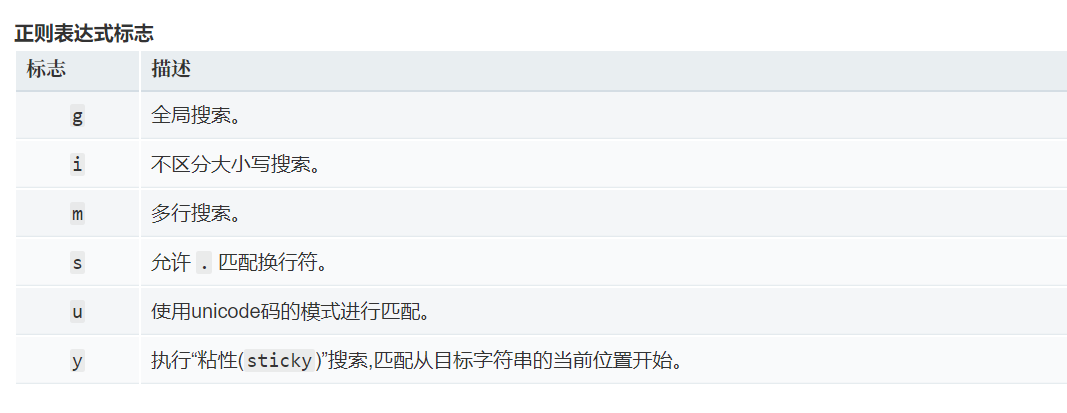### js正则表达式的实例化

• 第一种方法

var myRe = /d(b+)d/g;

• 第二种方法

var myRe = new RegExp("a\\w+d", "g");


### 使用正则表达式的方法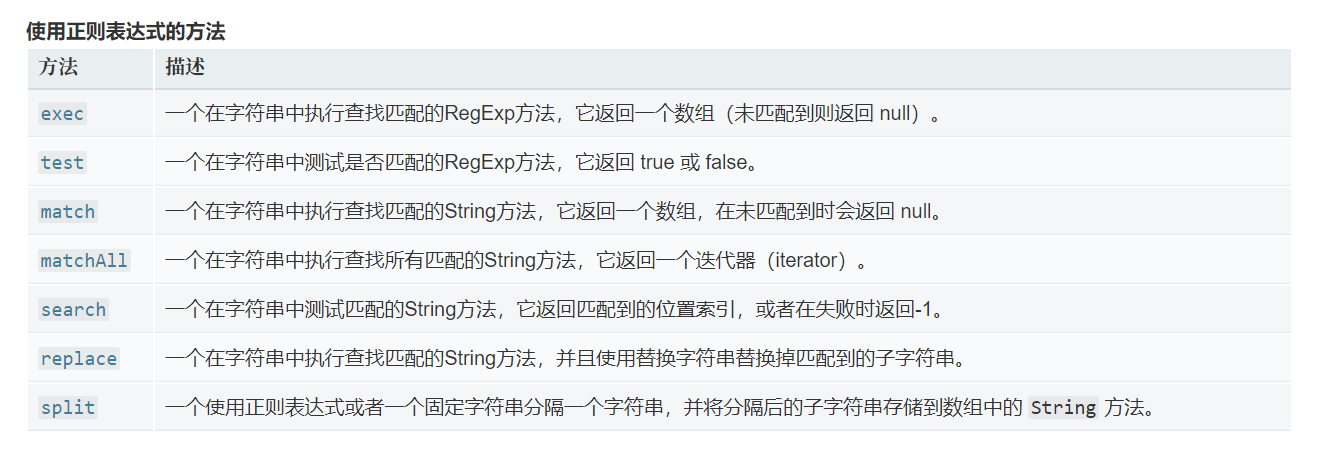matchAll() 方法返回一个包含所有匹配正则表达式的结果及分组捕获组的迭代器。

matchAll用法

const regexp = RegExp('foo[a-z]*','g');        const str = 'table football, foosball';        const matches = str.matchAll(regexp);        for (const match of matches) {            console.log(match);        }


# 正则案例集合分析

## JavaScript

posted @ 2020-08-31 11:49  sogeisetsu  阅读(162)  评论(0编辑  收藏  举报